Research papers and code for "Chao Gao":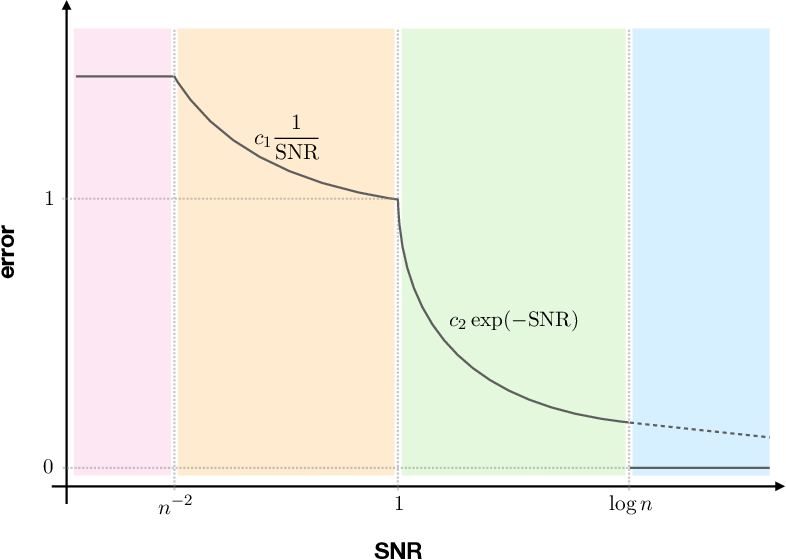We study the problem of approximate ranking from observations of pairwise interactions. The goal is to estimate the underlying ranks of $n$ objects from data through interactions of comparison or collaboration. Under a general framework of approximate ranking models, we characterize the exact optimal statistical error rates of estimating the underlying ranks. We discover important phase transition boundaries of the optimal error rates. Depending on the value of the signal-to-noise ratio (SNR) parameter, the optimal rate, as a function of SNR, is either trivial, polynomial, exponential or zero. The four corresponding regimes thus have completely different error behaviors. To the best of our knowledge, this phenomenon, especially the phase transition between the polynomial and the exponential rates, has not been discovered before.

Click to Read Paper and Get Code
This paper studies robust regression in the settings of Huber's $\epsilon$-contamination models. We consider estimators that are maximizers of multivariate regression depth functions. These estimators are shown to achieve minimax rates in the settings of $\epsilon$-contamination models for various regression problems including nonparametric regression, sparse linear regression, reduced rank regression, etc. We also discuss a general notion of depth function for linear operators that has potential applications in robust functional linear regression.

Click to Read Paper and Get Code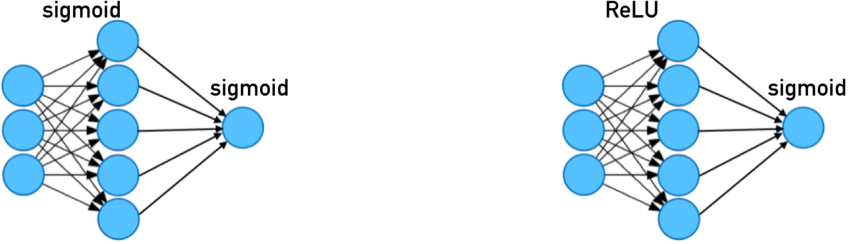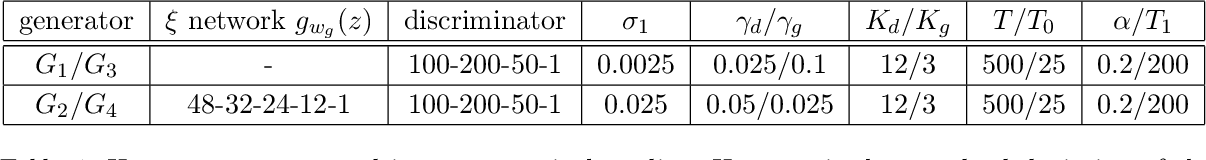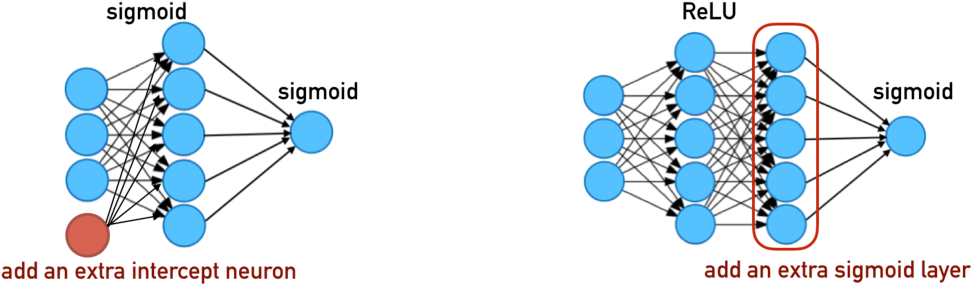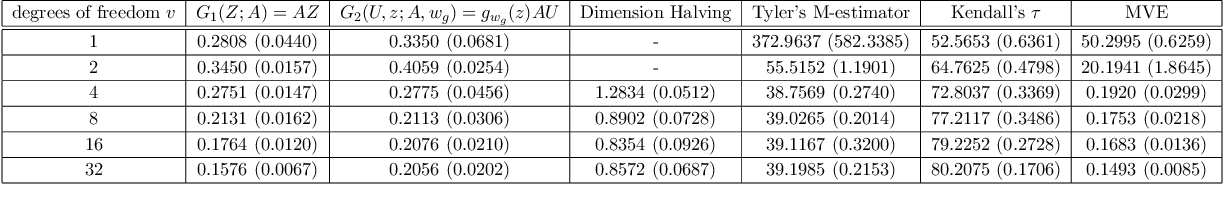Robust scatter estimation is a fundamental task in statistics. The recent discovery on the connection between robust estimation and generative adversarial nets (GANs) by Gao et al. (2018) suggests that it is possible to compute depth-like robust estimators using similar techniques that optimize GANs. In this paper, we introduce a general learning via classification framework based on the notion of proper scoring rules. This framework allows us to understand both matrix depth function and various GANs through the lens of variational approximations of $f$-divergences induced by proper scoring rules. We then propose a new class of robust scatter estimators in this framework by carefully constructing discriminators with appropriate neural network structures. These estimators are proved to achieve the minimax rate of scatter estimation under Huber's contamination model. Our numerical results demonstrate its good performance under various settings against competitors in the literature.

Click to Read Paper and Get Code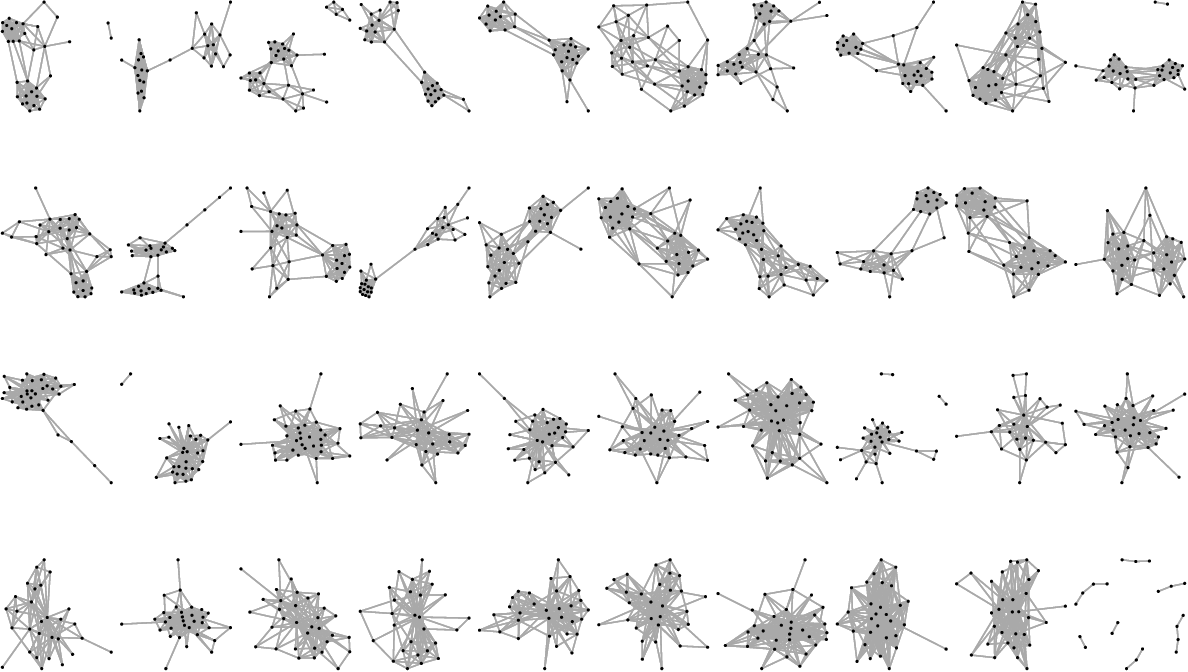This paper surveys some recent developments in fundamental limits and optimal algorithms for network analysis. We focus on minimax optimal rates in three fundamental problems of network analysis: graphon estimation, community detection, and hypothesis testing. For each problem, we review state-of-the-art results in the literature followed by general principles behind the optimal procedures that lead to minimax estimation and testing. This allows us to connect problems in network analysis to other statistical inference problems from a general perspective.

Click to Read Paper and Get Code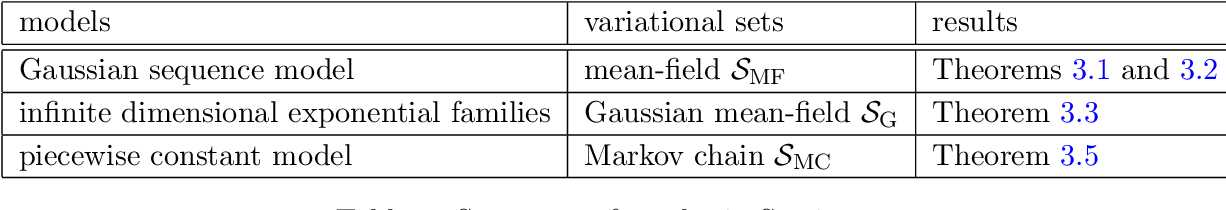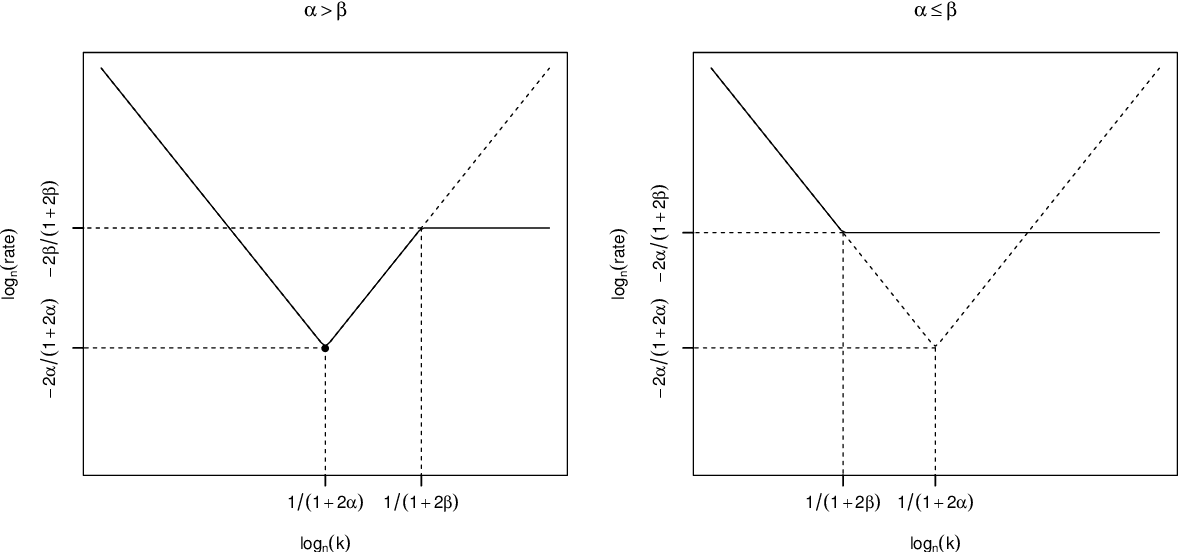We study convergence rates of variational posterior distributions for nonparametric and high-dimensional inference. We formulate general conditions on prior, likelihood, and variational class that characterize the convergence rates. Under similar "prior mass and testing" conditions considered in the literature, the rate is found to be the sum of two terms. The first term stands for the convergence rate of the true posterior distribution, and the second term is contributed by the variational approximation error. For a class of priors that admit the structure of a mixture of product measures, we propose a novel prior mass condition, under which the variational approximation error of the generalized mean-field class is dominated by convergence rate of the true posterior. We demonstrate the applicability of our general results for various models, prior distributions and variational classes by deriving convergence rates of the corresponding variational posteriors.

Click to Read Paper and Get Code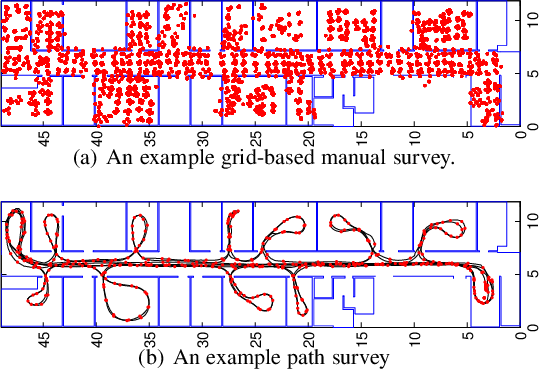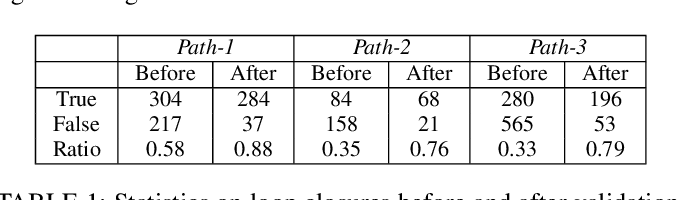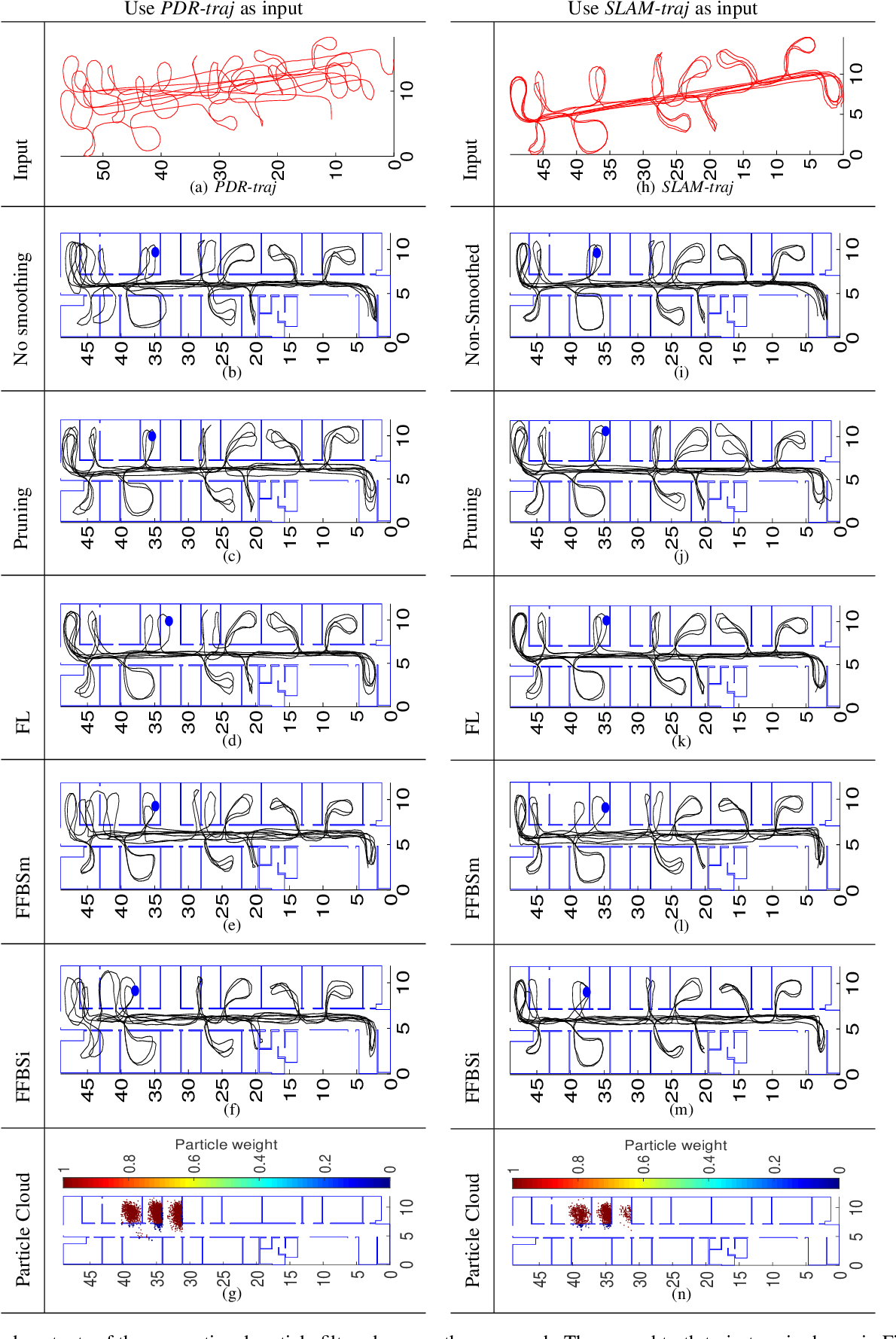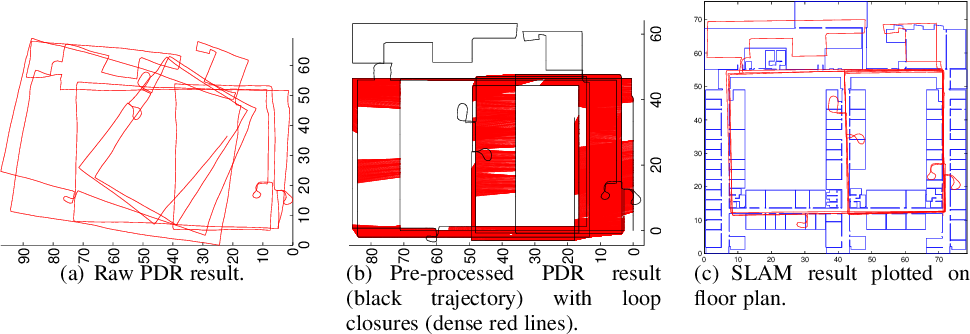Location fingerprinting locates devices based on pattern matching signal observations to a pre-defined signal map. This paper introduces a technique to enable fast signal map creation given a dedicated surveyor with a smartphone and floorplan. Our technique (PFSurvey) uses accelerometer, gyroscope and magnetometer data to estimate the surveyor's trajectory post-hoc using Simultaneous Localisation and Mapping and particle filtering to incorporate a building floorplan. We demonstrate conventional methods can fail to recover the survey path robustly and determine the room unambiguously. To counter this we use a novel loop closure detection method based on magnetic field signals and propose to incorporate the magnetic loop closures and straight-line constraints into the filtering process to ensure robust trajectory recovery. We show this allows room ambiguities to be resolved. An entire building can be surveyed by the proposed system in minutes rather than days. We evaluate in a large office space and compare to state-of-the-art approaches. We achieve trajectories within 1.1 m of the ground truth 90% of the time. Output signal maps well approximate those built from conventional, laborious manual survey. We also demonstrate that the signal maps built by PFSurvey provide similar or even better positioning performance than the manual signal maps.

Click to Read Paper and Get Code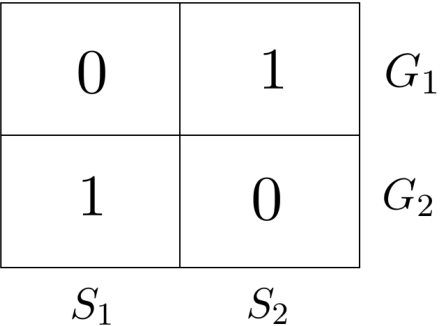Crowdsourcing has become a primary means for label collection in many real-world machine learning applications. A classical method for inferring the true labels from the noisy labels provided by crowdsourcing workers is Dawid-Skene estimator. In this paper, we prove convergence rates of a projected EM algorithm for the Dawid-Skene estimator. The revealed exponent in the rate of convergence is shown to be optimal via a lower bound argument. Our work resolves the long standing issue of whether Dawid-Skene estimator has sound theoretical guarantees besides its good performance observed in practice. In addition, a comparative study with majority voting illustrates both advantages and pitfalls of the Dawid-Skene estimator.

Click to Read Paper and Get Code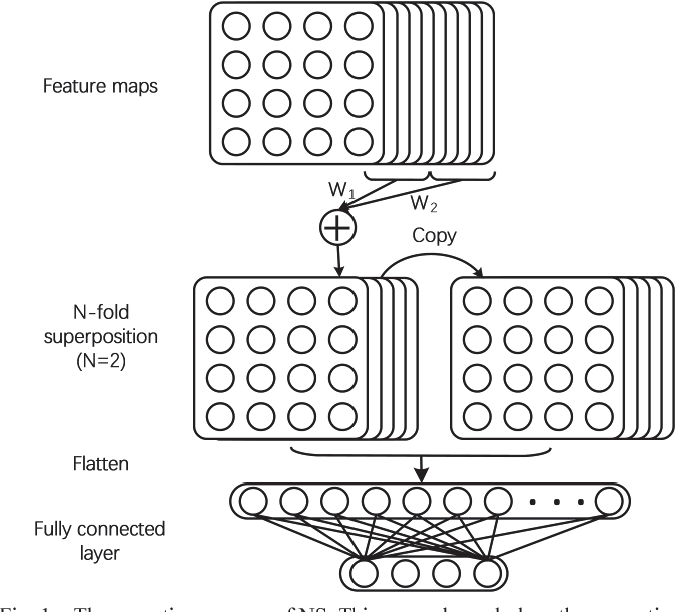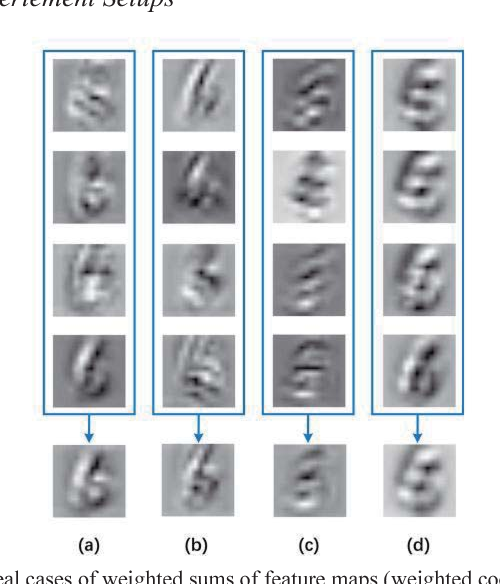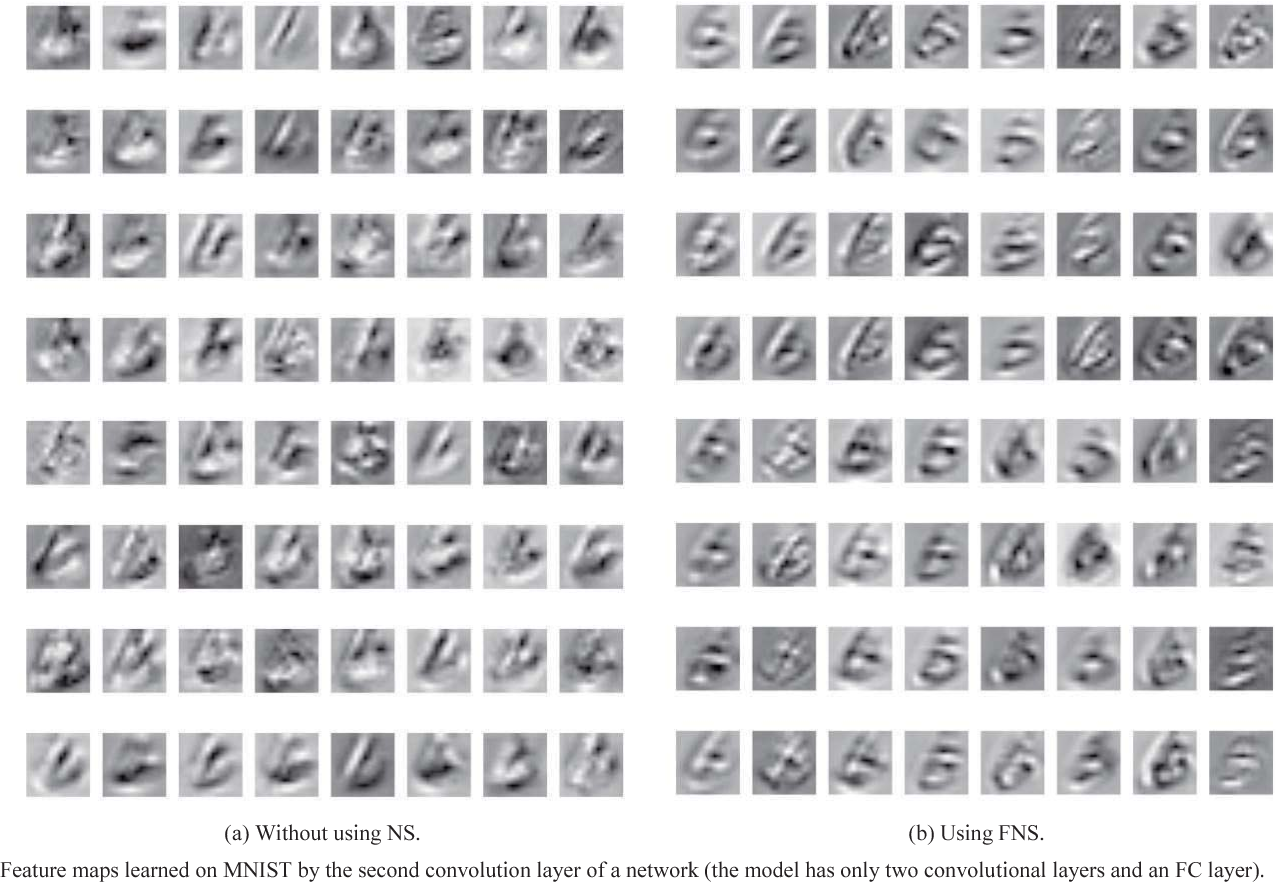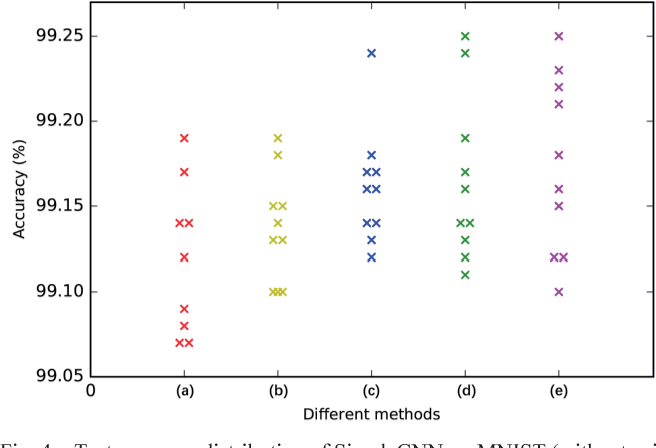Considering the use of Fully Connected (FC) layer limits the performance of Convolutional Neural Networks (CNNs), this paper develops a method to improve the coupling between the convolution layer and the FC layer by reducing the noise in Feature Maps (FMs). Our approach is divided into three steps. Firstly, we separate all the FMs into n blocks equally. Then, the weighted summation of FMs at the same position in all blocks constitutes a new block of FMs. Finally, we replicate this new block into n copies and concatenate them as the input to the FC layer. This sharing of FMs could reduce the noise in them apparently and avert the impact by a particular FM on the specific part weight of hidden layers, hence preventing the network from overfitting to some extent. Using the Fermat Lemma, we prove that this method could make the global minima value range of the loss function wider, by which makes it easier for neural networks to converge and accelerates the convergence process. This method does not significantly increase the amounts of network parameters (only a few more coefficients added), and the experiments demonstrate that this method could increase the convergence speed and improve the classification performance of neural networks.

* 7 pages, 5 figures, submitted to ICALIP 2018
Click to Read Paper and Get Code
In many machine learning applications, crowdsourcing has become the primary means for label collection. In this paper, we study the optimal error rate for aggregating labels provided by a set of non-expert workers. Under the classic Dawid-Skene model, we establish matching upper and lower bounds with an exact exponent $mI(\pi)$ in which $m$ is the number of workers and $I(\pi)$ the average Chernoff information that characterizes the workers' collective ability. Such an exact characterization of the error exponent allows us to state a precise sample size requirement $m>\frac{1}{I(\pi)}\log\frac{1}{\epsilon}$ in order to achieve an $\epsilon$ misclassification error. In addition, our results imply the optimality of various EM algorithms for crowdsourcing initialized by consistent estimators.

* To appear in the Proceedings of the 33rd International Conference on Machine Learning, New York, NY, USA, 2016
Click to Read Paper and Get Code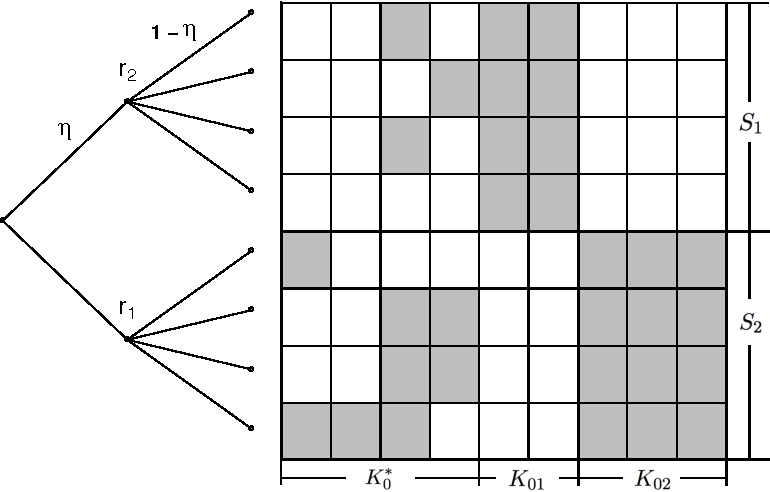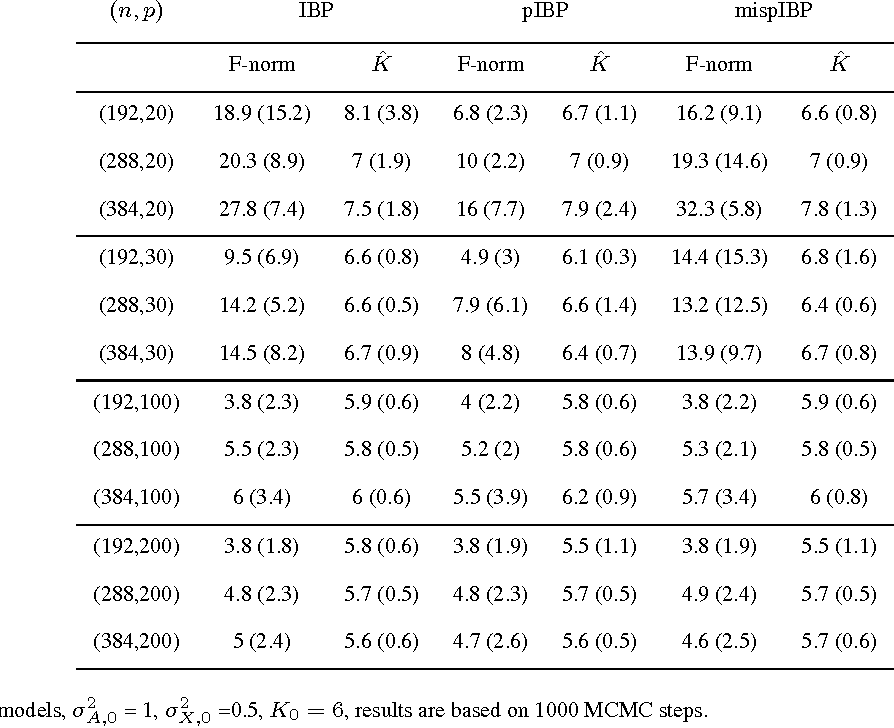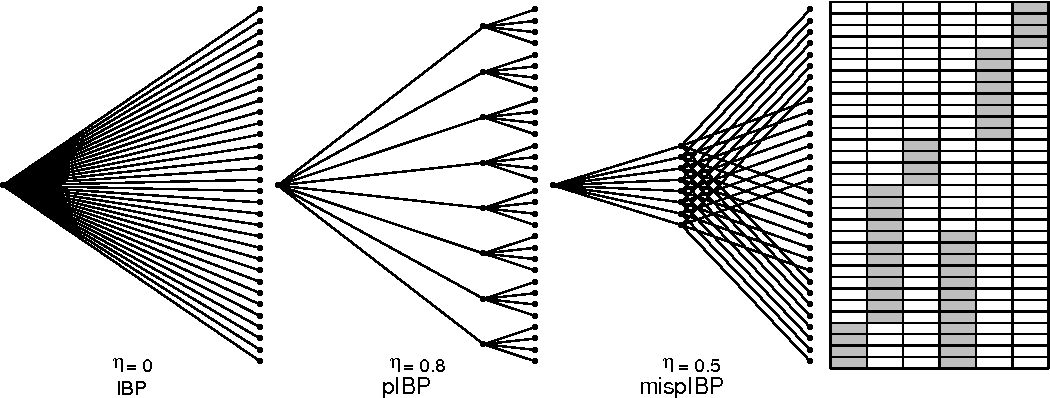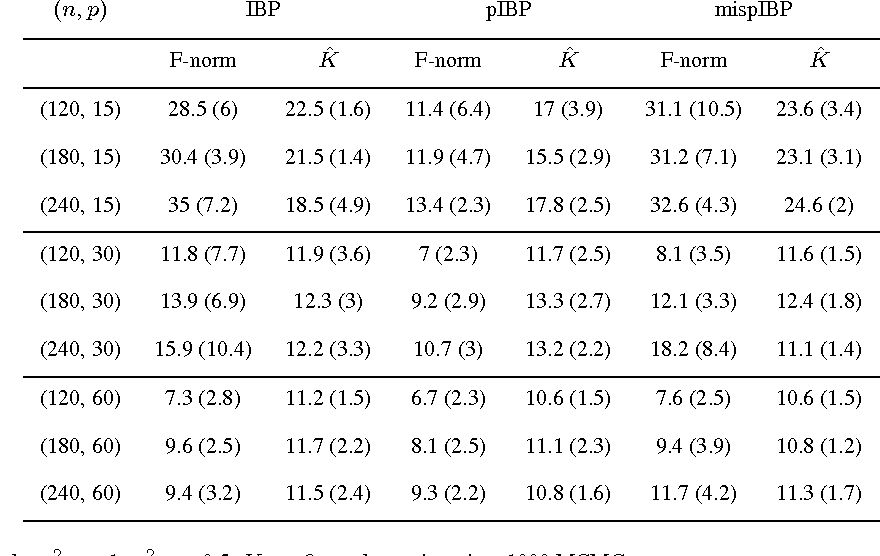By expressing prior distributions as general stochastic processes, nonparametric Bayesian methods provide a flexible way to incorporate prior knowledge and constrain the latent structure in statistical inference. The Indian buffet process (IBP) is such an example that can be used to define a prior distribution on infinite binary features, where the exchangeability among subjects is assumed. The phylogenetic Indian buffet process (pIBP), a derivative of IBP, enables the modeling of non-exchangeability among subjects through a stochastic process on a rooted tree, which is similar to that used in phylogenetics, to describe relationships among the subjects. In this paper, we study the theoretical properties of IBP and pIBP under a binary factor model. We establish the posterior contraction rates for both IBP and pIBP and substantiate the theoretical results through simulation studies. This is the first work addressing the frequentist property of the posterior behaviors of IBP and pIBP. We also demonstrated its practical usefulness by applying pIBP prior to a real data example arising in the field of cancer genomics where the exchangeability among subjects is violated.

Click to Read Paper and Get Code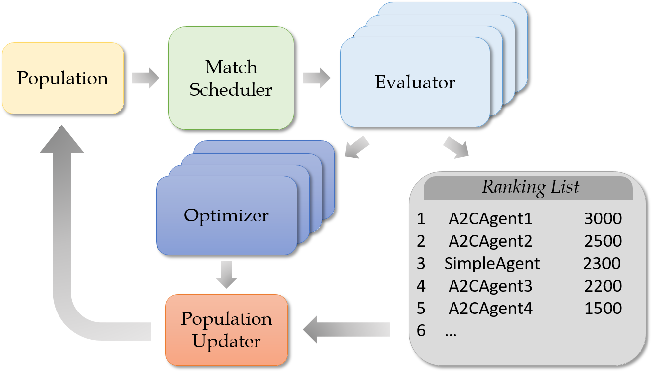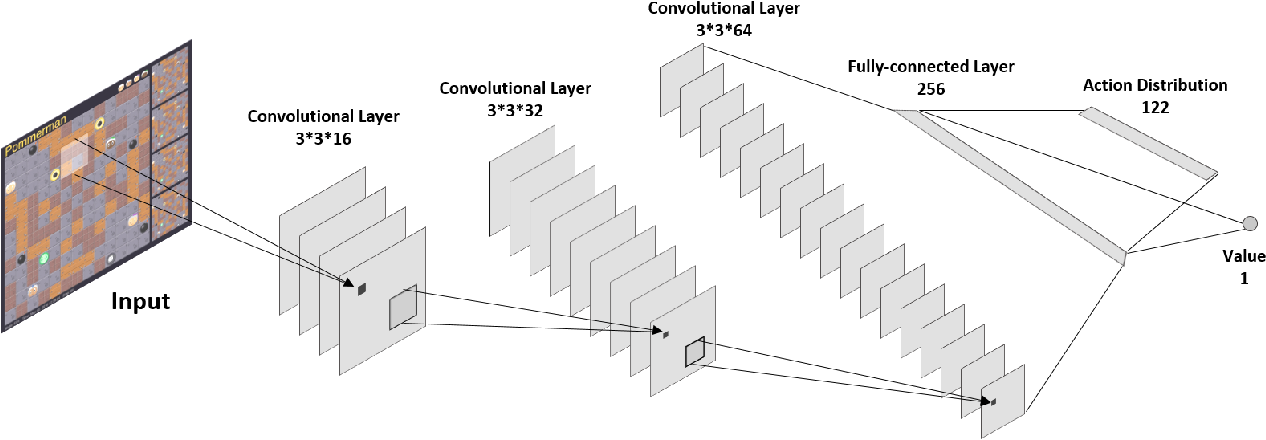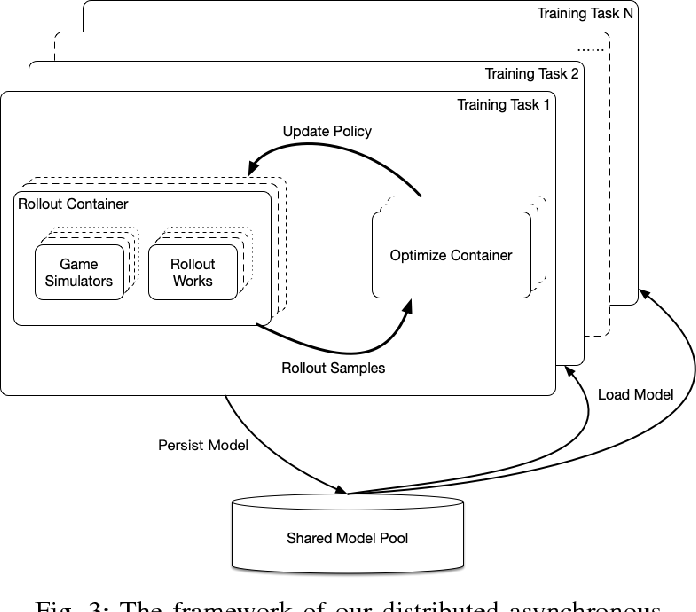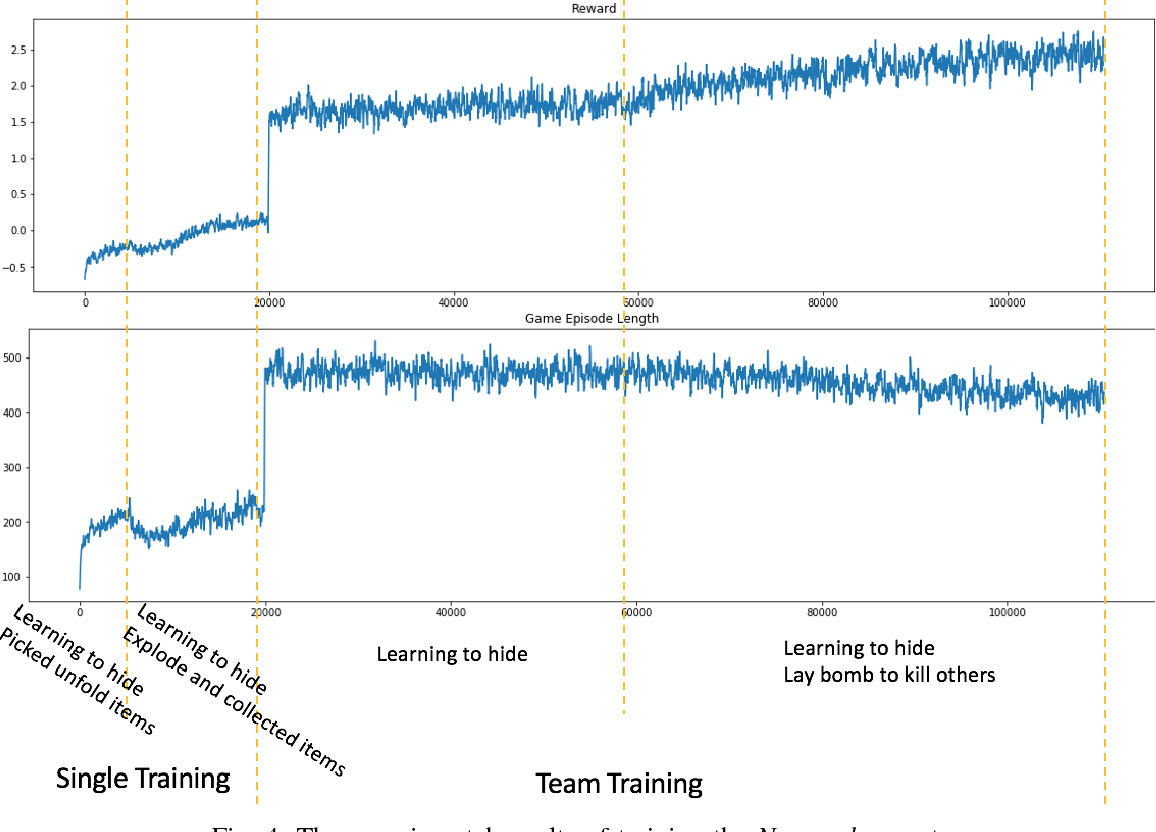Continual learning is the ability of agents to improve their capacities throughout multiple tasks continually. While recent works in the literature of continual learning mostly focused on developing either particular loss functions or specialized structures of neural network explaining the episodic memory or neural plasticity, we study continual learning from the perspective of the training mechanism. Specifically, we propose a COnitnual Match BAsed Training (COMBAT) framework for training a population of advantage-actor-critic (A2C) agents in Pommerman, a partially observable multi-agent environment with no communication. Following the COMBAT framework, we trained an agent, namely, Navocado, that won the title of the top 1 learning agent in the NeurIPS 2018 Pommerman Competition. Two critical features of our agent are worth mentioning. Firstly, our agent did not learn from any demonstrations. Secondly, our agent is highly reproducible. As a technical report, we articulate the design of state space, action space, reward, and most importantly, the COMBAT framework for our Pommerman agent. We show in the experiments that Pommerman is a perfect environment for studying continual learning, and the agent can improve its performance by continually learning new skills without forgetting the old ones. Finally, the result in the Pommerman Competition verifies the robustness of our agent when competing with various opponents.

* 8 pages, 7 figures
Click to Read Paper and Get Code
Recovering intrinsic low dimensional subspaces from data distributed on them is a key preprocessing step to many applications. In recent years, there has been a lot of work that models subspace recovery as low rank minimization problems. We find that some representative models, such as Robust Principal Component Analysis (R-PCA), Robust Low Rank Representation (R-LRR), and Robust Latent Low Rank Representation (R-LatLRR), are actually deeply connected. More specifically, we discover that once a solution to one of the models is obtained, we can obtain the solutions to other models in closed-form formulations. Since R-PCA is the simplest, our discovery makes it the center of low rank subspace recovery models. Our work has two important implications. First, R-PCA has a solid theoretical foundation. Under certain conditions, we could find better solutions to these low rank models at overwhelming probabilities, although these models are non-convex. Second, we can obtain significantly faster algorithms for these models by solving R-PCA first. The computation cost can be further cut by applying low complexity randomized algorithms, e.g., our novel $\ell_{2,1}$ filtering algorithm, to R-PCA. Experiments verify the advantages of our algorithms over other state-of-the-art ones that are based on the alternating direction method.

* Submitted to Neural Computation
Click to Read Paper and Get Code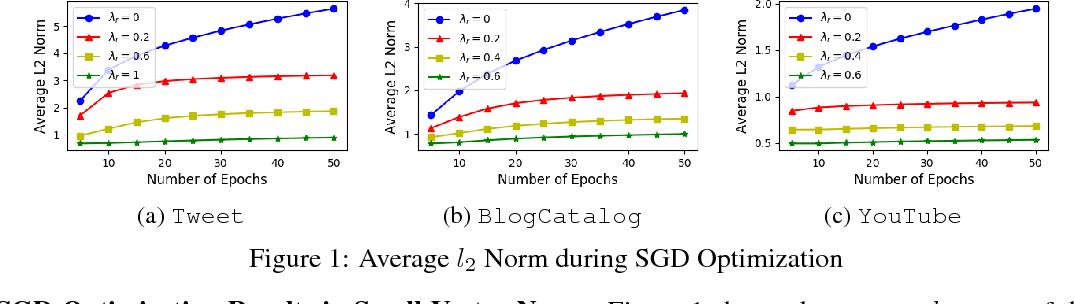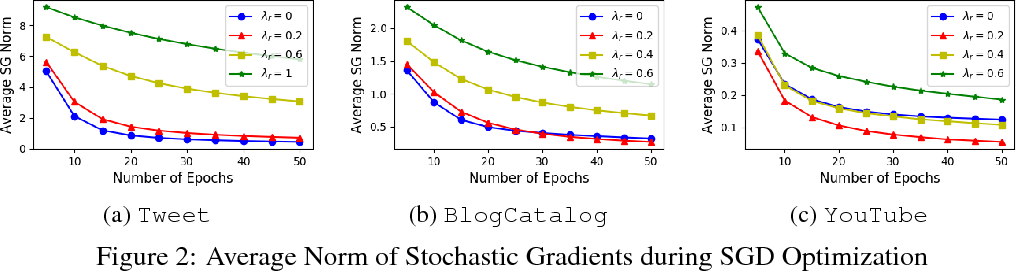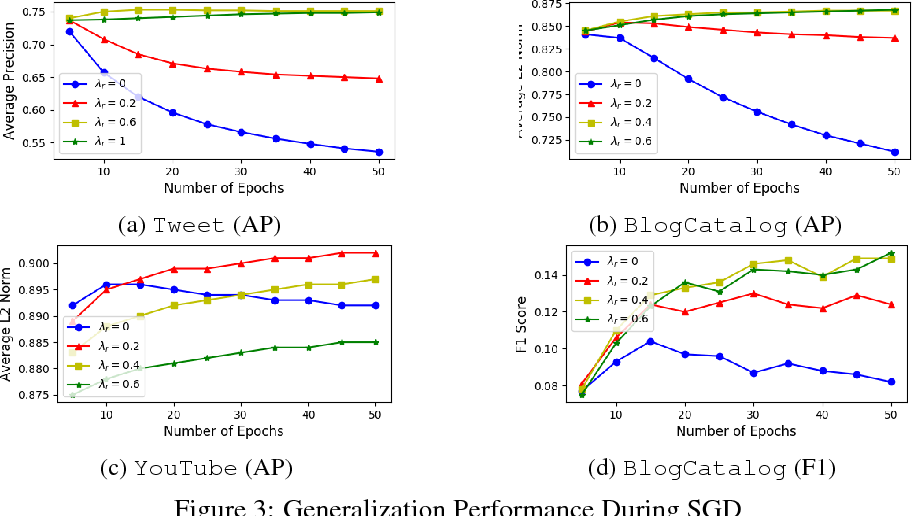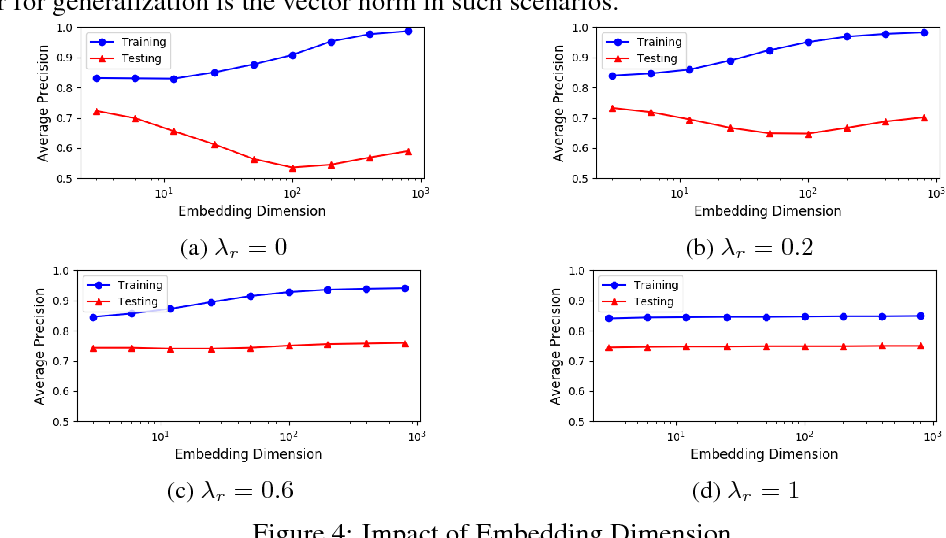Learning distributed representations for nodes in graphs is a crucial primitive in network analysis with a wide spectrum of applications. Linear graph embedding methods learn such representations by optimizing the likelihood of both positive and negative edges while constraining the dimension of the embedding vectors. We argue that the generalization performance of these methods is not due to the dimensionality constraint as commonly believed, but rather the small norm of embedding vectors. Both theoretical and empirical evidence are provided to support this argument: (a) we prove that the generalization error of these methods can be bounded by limiting the norm of vectors, regardless of the embedding dimension; (b) we show that the generalization performance of linear graph embedding methods is correlated with the norm of embedding vectors, which is small due to the early stopping of SGD and the vanishing gradients. We performed extensive experiments to validate our analysis and showcased the importance of proper norm regularization in practice.

Click to Read Paper and Get Code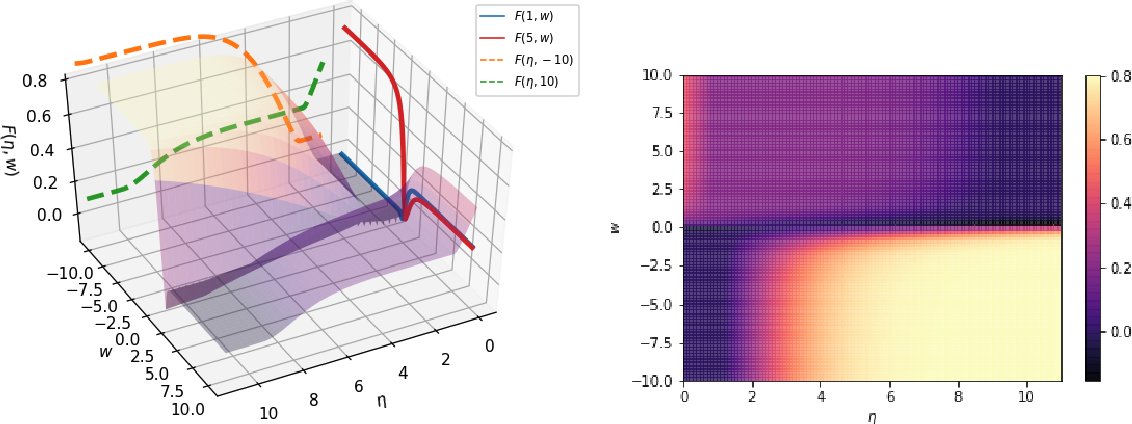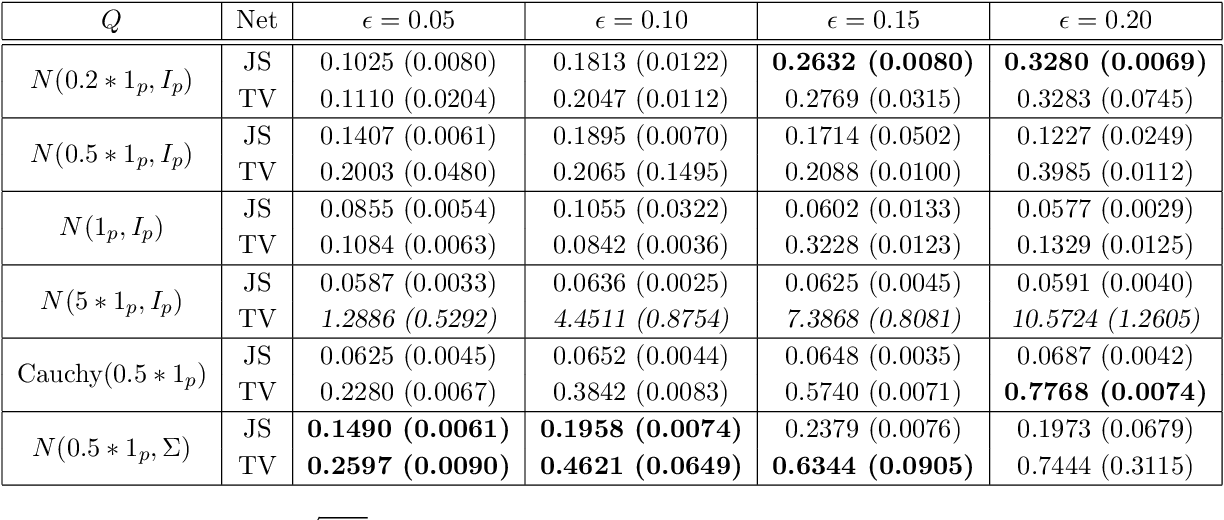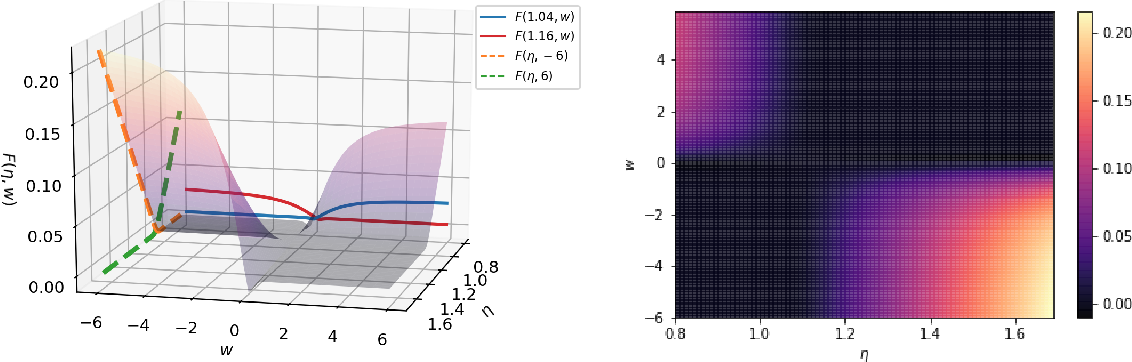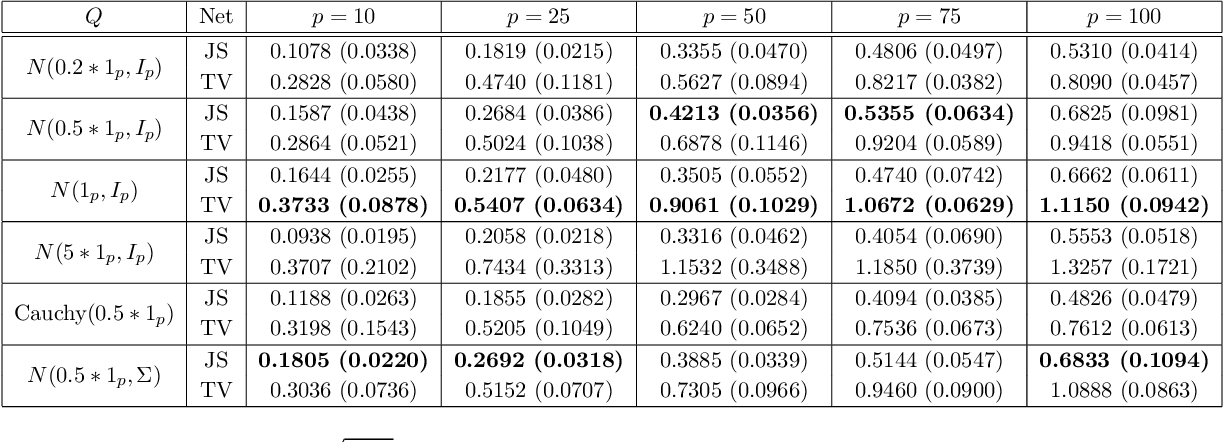Robust estimation under Huber's $\epsilon$-contamination model has become an important topic in statistics and theoretical computer science. Rate-optimal procedures such as Tukey's median and other estimators based on statistical depth functions are impractical because of their computational intractability. In this paper, we establish an intriguing connection between f-GANs and various depth functions through the lens of f-Learning. Similar to the derivation of f-GAN, we show that these depth functions that lead to rate-optimal robust estimators can all be viewed as variational lower bounds of the total variation distance in the framework of f-Learning. This connection opens the door of computing robust estimators using tools developed for training GANs. In particular, we show that a JS-GAN that uses a neural network discriminator with at least one hidden layer is able to achieve the minimax rate of robust mean estimation under Huber's $\epsilon$-contamination model. Interestingly, the hidden layers for the neural net structure in the discriminator class is shown to be necessary for robust estimation.

Click to Read Paper and Get Code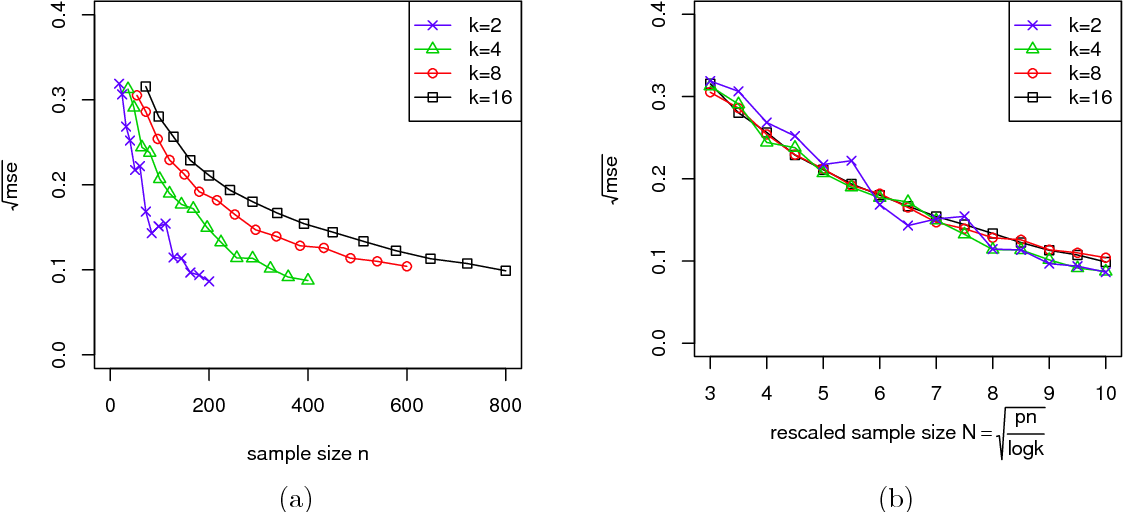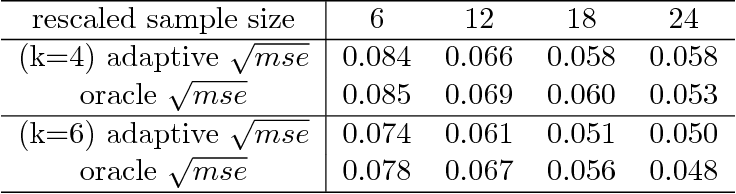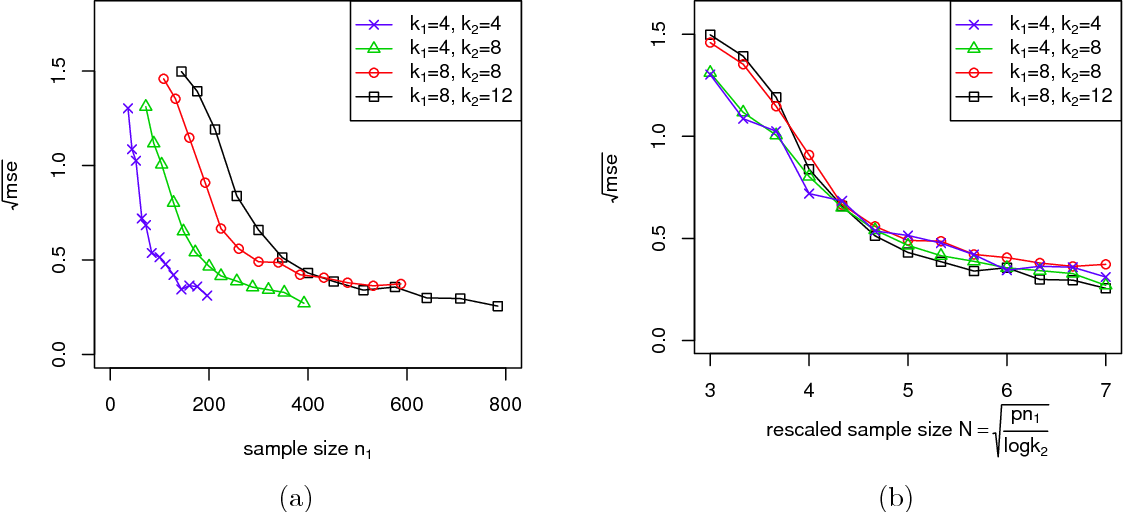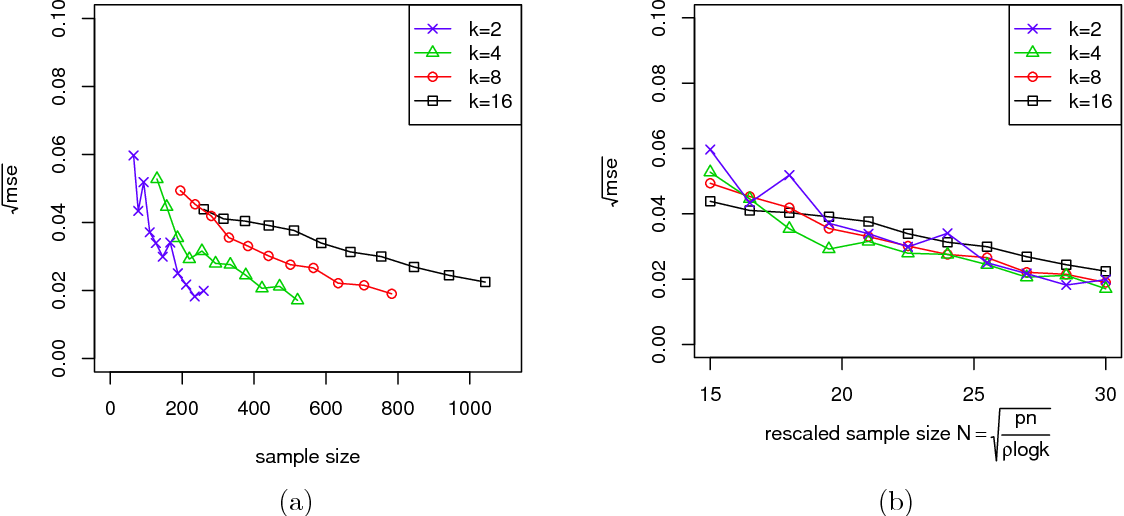Biclustering structures in data matrices were first formalized in a seminal paper by John Hartigan (1972) where one seeks to cluster cases and variables simultaneously. Such structures are also prevalent in block modeling of networks. In this paper, we develop a unified theory for the estimation and completion of matrices with biclustering structures, where the data is a partially observed and noise contaminated data matrix with a certain biclustering structure. In particular, we show that a constrained least squares estimator achieves minimax rate-optimal performance in several of the most important scenarios. To this end, we derive unified high probability upper bounds for all sub-Gaussian data and also provide matching minimax lower bounds in both Gaussian and binary cases. Due to the close connection of graphon to stochastic block models, an immediate consequence of our general results is a minimax rate-optimal estimator for sparse graphons.

Click to Read Paper and Get Code
We tightly analyze the sample complexity of CCA, provide a learning algorithm that achieves optimal statistical performance in time linear in the required number of samples (up to log factors), as well as a streaming algorithm with similar guarantees.

Click to Read Paper and Get Code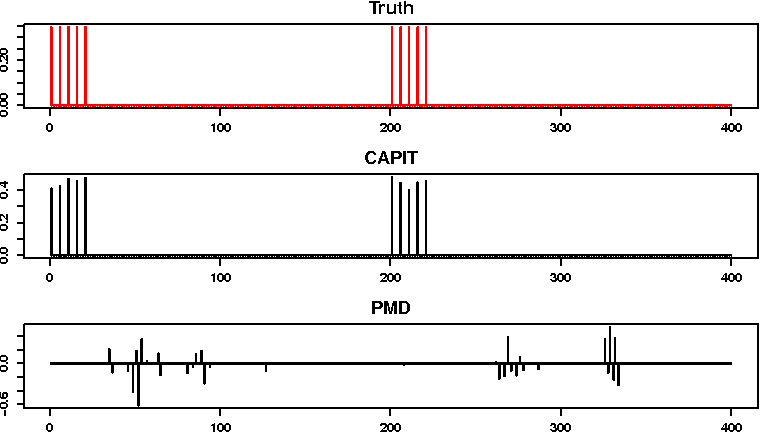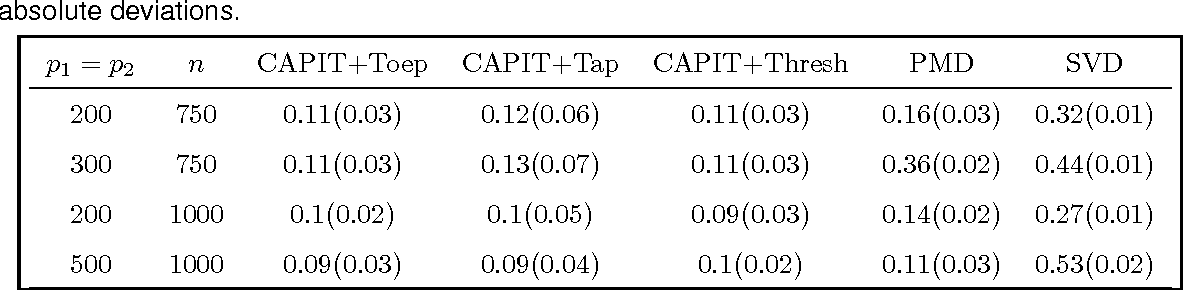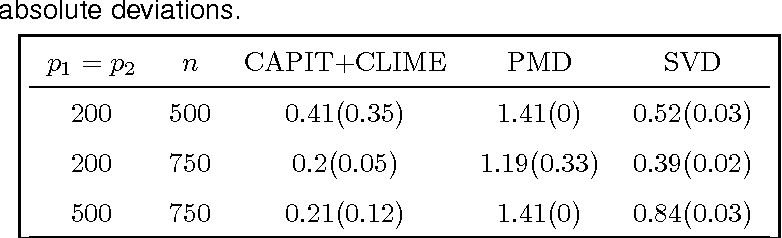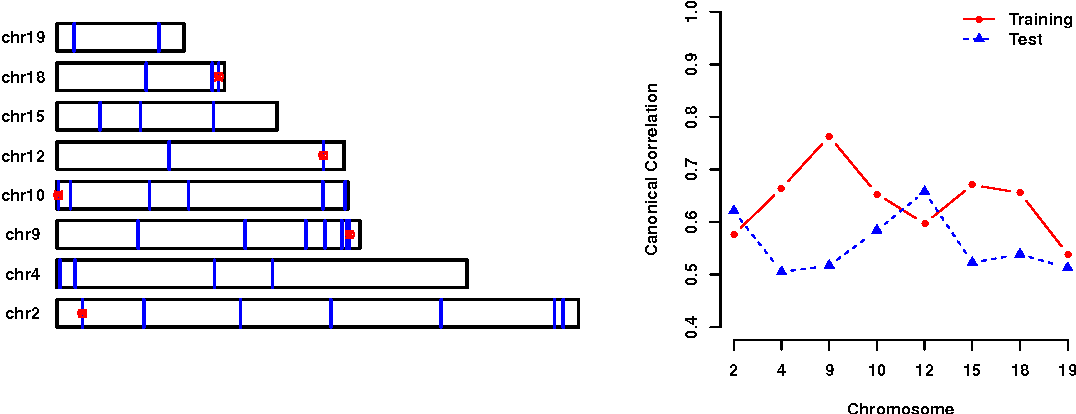Sparse Canonical Correlation Analysis (CCA) has received considerable attention in high-dimensional data analysis to study the relationship between two sets of random variables. However, there has been remarkably little theoretical statistical foundation on sparse CCA in high-dimensional settings despite active methodological and applied research activities. In this paper, we introduce an elementary sufficient and necessary characterization such that the solution of CCA is indeed sparse, propose a computationally efficient procedure, called CAPIT, to estimate the canonical directions, and show that the procedure is rate-optimal under various assumptions on nuisance parameters. The procedure is applied to a breast cancer dataset from The Cancer Genome Atlas project. We identify methylation probes that are associated with genes, which have been previously characterized as prognosis signatures of the metastasis of breast cancer.

Click to Read Paper and Get Code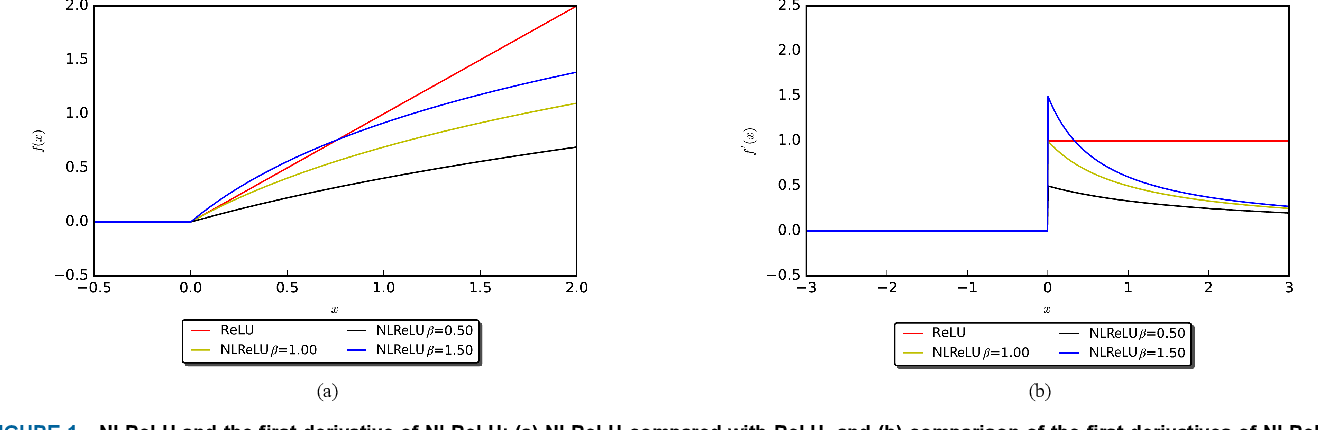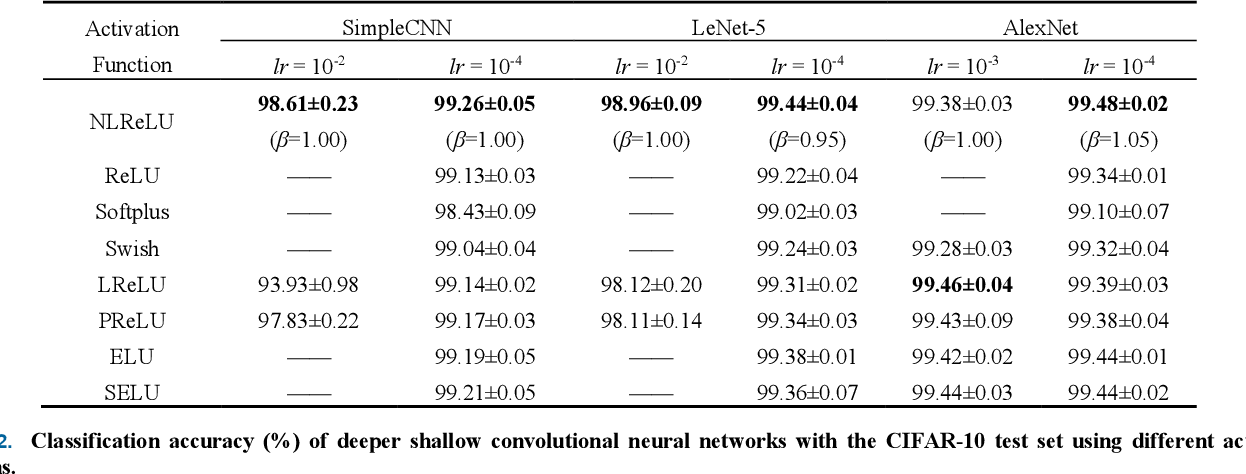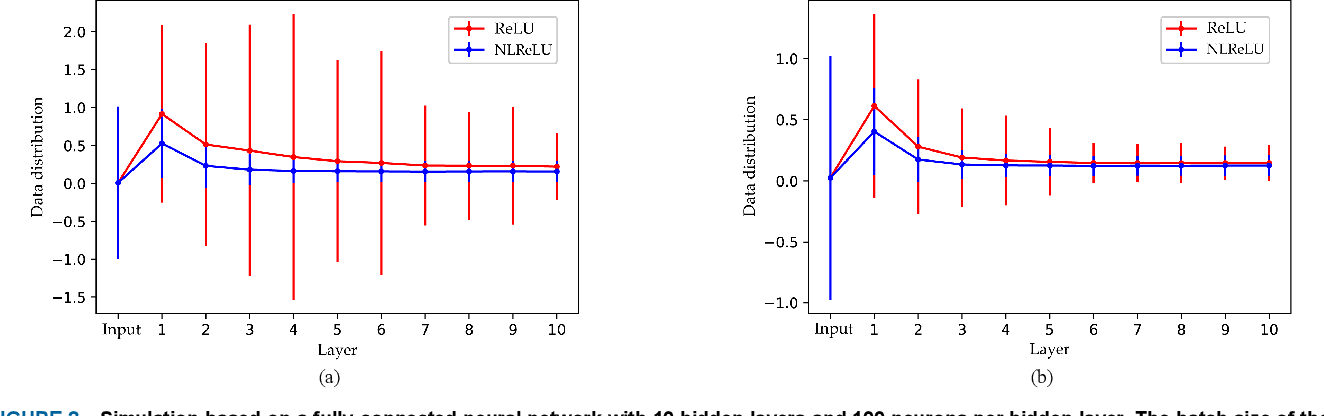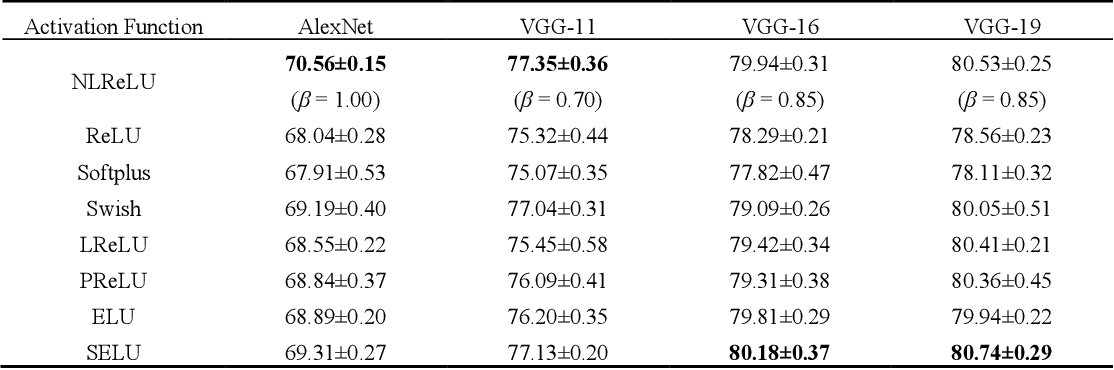Activation functions play a key role in providing remarkable performance in deep neural networks, and the rectified linear unit (ReLU) is one of the most widely used activation functions. Various new activation functions and improvements on ReLU have been proposed, but each carry performance drawbacks. In this paper, we propose an improved activation function, which we name the natural-logarithm-rectified linear unit (NLReLU). This activation function uses the parametric natural logarithmic transform to improve ReLU and is simply defined as. NLReLU not only retains the sparse activation characteristic of ReLU, but it also alleviates the "dying ReLU" and vanishing gradient problems to some extent. It also reduces the bias shift effect and heteroscedasticity of neuron data distributions among network layers in order to accelerate the learning process. The proposed method was verified across ten convolutional neural networks with different depths for two essential datasets. Experiments illustrate that convolutional neural networks with NLReLU exhibit higher accuracy than those with ReLU, and that NLReLU is comparable to other well-known activation functions. NLReLU provides 0.16% and 2.04% higher classification accuracy on average compared to ReLU when used in shallow convolutional neural networks with the MNIST and CIFAR-10 datasets, respectively. The average accuracy of deep convolutional neural networks with NLReLU is 1.35% higher on average with the CIFAR-10 dataset.

Click to Read Paper and Get Code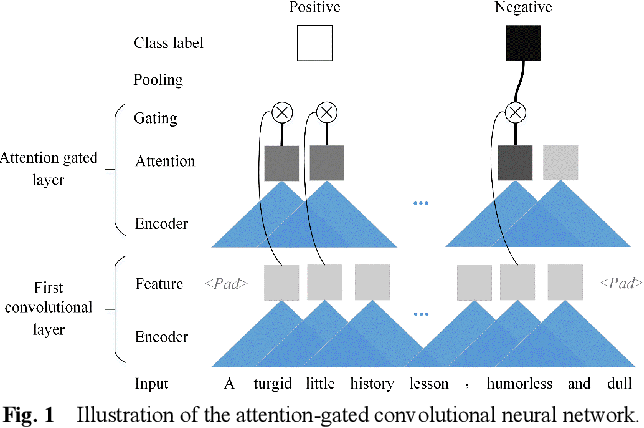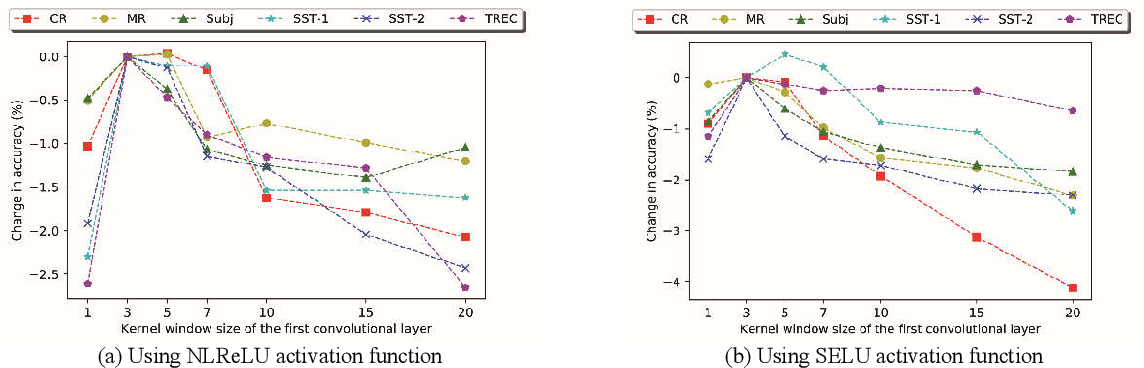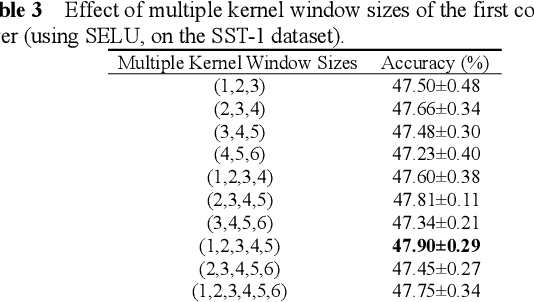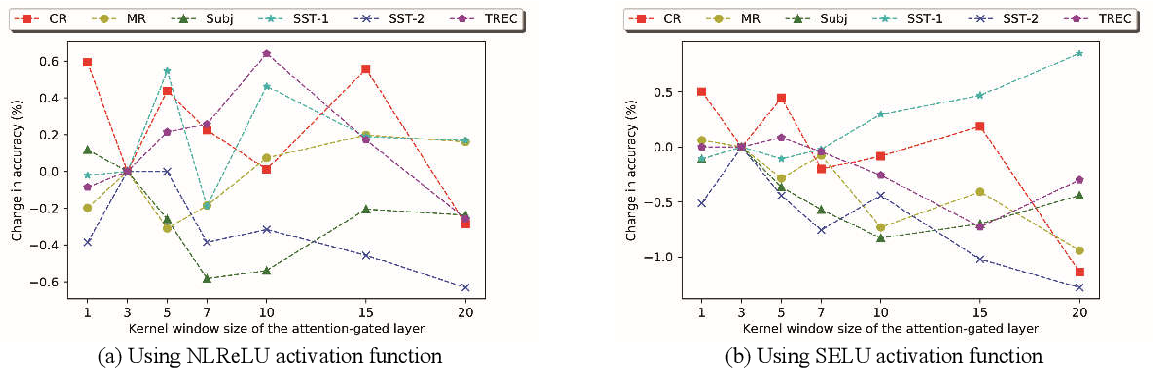Recently, Attention-Gated Convolutional Neural Networks (AGCNNs) perform well on several essential sentence classification tasks and show robust performance in practical applications. However, AGCNNs are required to set many hyperparameters, and it is not known how sensitive the model's performance changes with them. In this paper, we conduct a sensitivity analysis on the effect of different hyperparameters s of AGCNNs, e.g., the kernel window size and the number of feature maps. Also, we investigate the effect of different combinations of hyperparameters settings on the model's performance to analyze to what extent different parameters settings contribute to AGCNNs' performance. Meanwhile, we draw practical advice from a wide range of empirical results. Through the sensitivity analysis experiment, we improve the hyperparameters settings of AGCNNs. Experiments show that our proposals achieve an average of 0.81% and 0.67% improvements on AGCNN-NLReLU-rand and AGCNN-SELU-rand, respectively; and an average of 0.47% and 0.45% improvements on AGCNN-NLReLU-static and AGCNN-SELU-static, respectively.

Click to Read Paper and Get Code